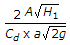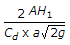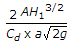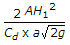# Mechanical Engineering - Hydraulics and Fluid Mechanics - Discussion

37.

A tank of uniform cross-sectional area (A) containing liquid upto height (H1) has an orifice of cross-sectional area (a) at its bottom. The time required to empty the tank completely will be

 [A].[B].[C].[D].Explanation:

No answer description available for this question.

 Nihal said: (Jan 16, 2015) It should be. Volume/(Discharge). = AH/(Cd*a*(2gH)^.5). = (A*(H)^.5)/(Cd*a*(2g)^.5). Why is there an extra 2 in the numerator?

 Heera Lal Choudhary said: (Jun 22, 2016) Why is there extra 2?

 Ashutosh Gupta said: (Jul 19, 2016) Because the above formula is applicable for a particular height say H1. As the water level decreases speed of flow will also decrease from max to minimum (zero) hence the average.

 Niladri Shree Chandan said: (Aug 26, 2016) Q = a * Velocity = A * (dh/dt). => a * (2gh)^0.5 = A * (dh/dt). => a * (2g)^0.5 * dt = A * (dh/(h^0.5)). Integrating above gives the result.

 Dn Bhaskar said: (Dec 8, 2017) Right @Ashutosh.

 Ayush said: (Oct 5, 2019) Let at time t height of water in container is h and after time dt, dh height of water flows out. At this postition dQ/dt = sqrt(2gh)*a. A*dh=sqrt(2gh)*a*dt. Integrating this with lower limit 0 and higher limit H we will get the answer.